` `

# Vector menu¶

Vector menu provides a one-stop resource for many common vector-based GIS tasks. It provides a growing suite of spatial data management and analysis functions that are both fast and functional. These features are part of the processing framework. Note that some plugins can add other features so, in this chapter, only default features from processing framework will be listed.

Processing framework is automatically installed and, like any plugin, can be enabled and disabled using the Plugin Manager (see The Plugins Dialog). When enabled, the Processing plugin adds a Vector menu to QGIS, providing functions ranging from Analysis and Research Tools to Geometry and Geoprocessing Tools, as well as several useful Data Management Tools.

See QGIS processing framework for more information about the processing framework.

## Analysis tools¶

Icon Tool Purpose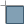Distance matrix Measure distances between two point layers, and output results as a) Square distance matrix, b) Linear distance matrix, or c) Summary of distances. Can limit distances to the k nearest features.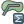Sum line length Calculate the total sum of line lengths for each polygon of a polygon vector layer.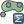Points in polygon Count the number of points that occur in each polygon of an input polygon vector layer.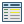List unique values List all unique values in an input vector layer field.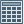Basic statistics for text field Compute basic statistics (mean, std dev, N, sum, CV) on an input text field.Basic statistics for numeric field Compute basic statistics (mean, std dev, N, sum, CV) on an input numeric field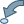Nearest neighbor analysis Compute nearest neighbor statistics to assess the level of clustering in a point vector layer.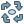Mean coordinate(s) Compute either the normal or weighted mean center of an entire vector layer, or multiple features based on a unique ID field.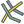Line intersections Locate intersections between lines, and output results as a point shapefile. Useful for locating road or stream intersections, ignores line intersections with length > 0.

Table tools 1: Default tools in Analysis group

## Research tools¶

Icon Tool Purpose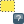Random selection Randomly select n number of features, or n percentage of features.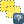Random selection within subsets Randomly select features within subsets based on a unique ID field.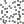Random points inside polygons Generate pseudo-random points over a polygon layer (variable number of point or fixed number of point).Random points in extent Generate pseudo-random points over a given extent.Random points in layer bounds Generate pseudo-random points over bounds of a given input layer.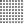Regular points Generate a regular grid of points over a specified region and export them as a point shapefile.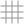Vector grid Generate a line or polygon grid based on user-specified grid spacing.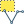Select by location Select features based on their location relative to another layer to form a new selection, or add or subtract from the current selection.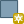Polygon from layer extent Create a single rectangular polygon layer from the extent of an input raster or vector layer.

Table Tools 2: Default tools in Research group

## Geoprocessing tools¶

Icon Tool Purpose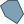Convex hull(s) Create minimum convex hull(s) for an input layer, or based on an ID field.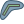Buffer with * fixed distance * distance field Create buffer(s) around features * based on fixed distance * based on distance field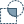Intersect Overlay layers such that output contains areas where both layers intersect.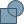Union Overlay layers such that output contains intersecting and non-intersecting areas.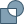Symmetrical difference Overlay layers such that output contains those areas of the input and difference layers that do not intersect.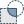Clip Overlay layers such that output contains areas that intersect the clip layer.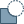Difference Overlay layers such that output contains areas not intersecting the clip layer.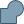Dissolve Merge features based on input field. All features with identical input values are combined to form one single feature.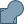Eliminate sliver polygons Merges selected features with the neighboring polygon with the largest area or largest common boundary.

Table Tools 3: Default tools in Geoprocessing group

## Geometry tools¶

Icon Tool Purpose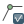Check geometry validity Check polygons for intersections, closed holes, and fix node ordering. You can choose the engine used by the in the options dialog, digitizing tab Change the Validate geometries value. There is two engines: QGIS and GEOS which have pretty different behavior. Another tools exists which shows different result as well: Topology Checker plugin and ‘must not have invalid geometries’ rule.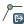Export/Add geometry columns Add vector layer geometry info to point (XCOORD, YCOORD), line (LENGTH), or polygon (AREA, PERIMETER) layer.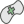Polygon centroids Calculate the true centroids for each polygon in an input polygon layer.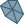Delaunay triangulation Calculate and output (as polygons) the Delaunay triangulation of an input point vector layer.
Voronoi polygons Calculate Voronoi polygons of an input point vector layer.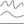Simplify geometry Generalize lines or polygons with a modified Douglas-Peucker algorithm.
Densify geometry Densify lines or polygons by adding vertices.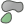Multipart to singleparts Convert multipart features to multiple singlepart features. Creates simple polygons and lines.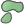Singleparts to multipart Merge multiple features to a single multipart feature based on a unique ID field.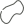Polygons to lines Convert polygons to lines, multipart polygons to multiple singlepart lines.Lines to polygons Convert lines to polygons, multipart lines to multiple singlepart polygons.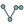Extract nodes Extract nodes from line and polygon layers and output them as points.

Table Tools 4: Default tools in Geometry group

Note

The Simplify geometry tool can be used to remove duplicate nodes in line and polygon geometries. Just set the Simplify tolerance parameter to 0 and this will do the trick.

## Data management tools¶

Icon Tool Purpose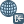Define current projection Specify the CRS for shapefiles whose CRS has not been defined.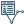Join attributes by location Join additional attributes to vector layer based on spatial relationship. Attributes from one vector layer are appended to the attribute table of another layer and exported as a shapefile.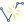Split vector layer Split input layer into multiple separate layers based on input field.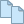Merge shapefiles to one Merge several shapefiles within a folder into a new shapefile based on the layer type (point, line, area).
Create spatial index Create a spatial index for OGR- supported formats.

Table Tools 5: Default tools in Data management group